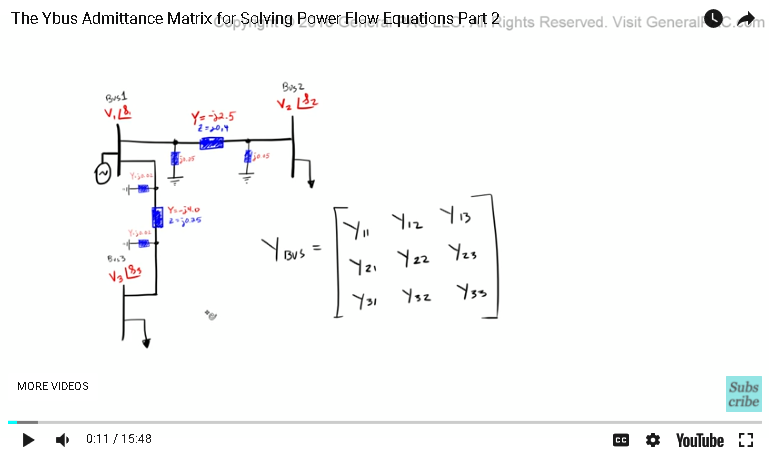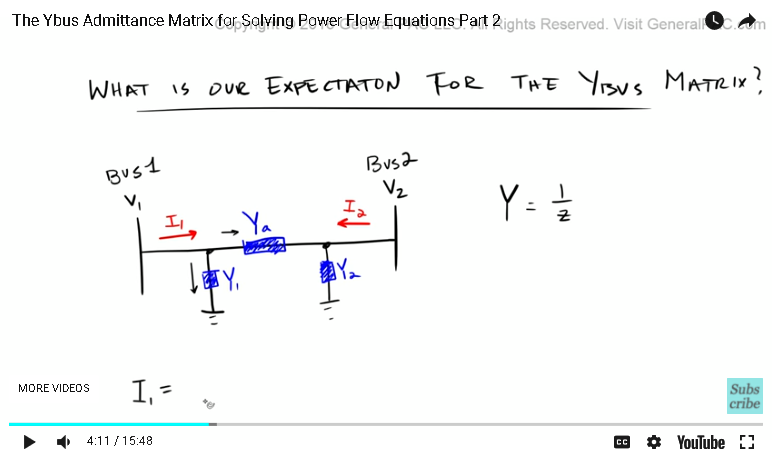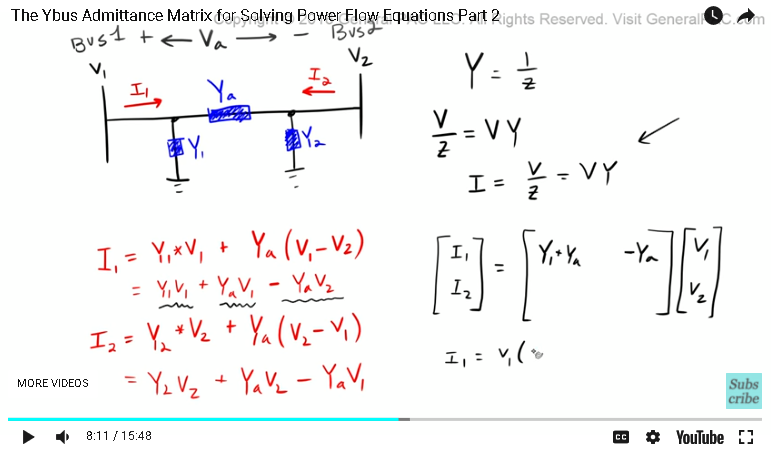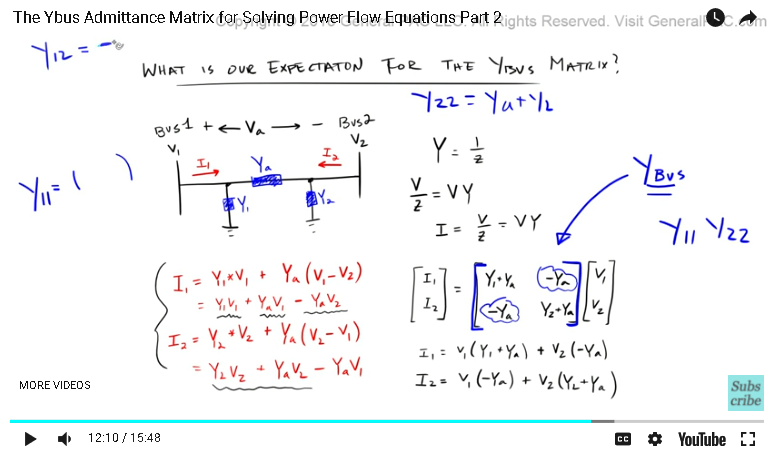This video was brought to you by GeneralPAC.com, making power systems Intuitive, Open and Free for Everyone, Everywhere. Consider subscribing and supporting through patreon.com/GeneralPAC. This is a mechanism for you to support us financially so we can continue making high quality power system video tutorials. Our corporate sponsor for this topic is AllumiaX.com from Seattle, Washington. Contact them for industrial and commercial power system studies.

A tutorial on the YBus admittance matrix for power flow equations, part two.

In part one, we took our time and drew this entire three Bus system and defined all of the impedances and calculated admittances for each of our transmission lines. We said that both of these transmission lines were medium length, which has shunt admittance to it, and these shunt admittances modeled by these two things. Okay?Now, another term for shunt admittance, that I didn't cover in the previous tutorial, is line charging capacitance. So when we say shunt admittance, we actually are talking about the line charging capacitance for both of these transmission lines. And then, another term to define this admittance here, or this impedance, is a series impedance or series admittance. Which makes sense because it's in series with Bus 1 and Bus 2.

Similarly, this admittance is also considered as a series impedance or a series admittance, and these two guys are your shunt admittance or line charging capacitance.

Before we into actually calculating this YBus, let's spend some time understanding exactly how we come up with the values in this particular matrix. Some of the terms to keep in mind, and this is very, very important, is that these terms right here, the middle terms or the diagonal terms, right? Y11, Y22, and Y33. These are called the diagonal admittances, okay? And then, everything else, which is these guys here, these are called out off-diagonal admittances.

So it's very, very important to keep this terminology straight. We're going to spend some time in answering this question, "What is our expectation for the YBus matrix?", and this is really important to understand. If we can understand what our expectation is for the YBus matrix, then we know exactly how it works. Let's try to answer this particular question.

We'll answer this question by drawing two Buses, right? Let's say that we have Bus1 and Bus2, then let's say we have a transmission line that connects Bus1 and Bus2, similar to the example above, we had some sort of admittance that models the admittance of the transmission line, and we also had some line capacitance or shunt admittance, which we'll model like this.

Okay, so we'll say that this admittance is equal to Ya, and this admittance was equal to Y1, this admittance was equal to Y2. And then we'll define current that's going this way, and then current that's coming out that way. Now, notice that I1 and I2 are in opposite directions, so they should equal the negative of each other, right? Then let's define V1 here and V2 there, okay? We have voltage for Bus1 and voltage for Bus2.

Okay, now remember, one thing to really keep in mind, is that Y, the admittance, is simply equal to 1 over Z, okay? Now, if you were to calculate what the current I1 was, with respect to the admittance of the transition line plus the shunt admittance of this particular transmission line, how would we do that?

Y1, okay, we know that Y1 ... that's going to equal the current that's going through this part of the line, right? Plus the current that is going into this part of the line. Okay, so Y1 is going to equal the admittance of Y1, right? Admittance of Y1 times that by the voltage of V1. Now, this makes sense, right? Because we've said that Y was equal to 1 over Z, so if we were to calculate the current going through this admittance here, well that's just equal to the voltage over the impedance, right? The voltage we already know, we already defined the voltage of V1, and the admittance is just 1 over Z, so that simply [inaudible 00:04:47] voltage x admittance, right? So this makes sense, so now we're going to add, + the current that's going into this admittance here.Well, this admittance is simply equal to the voltage drop between Bus1 and Bus2, so let's just call this voltage drop Va, and so we're going to define this voltage drop as like that. Now, similar to the first part, we have our admittance, Ya, right? Which is this admittance here, and we're going to multiply the voltage drop between Bus1 and Bus2, so that's simply going to equal V1 minus V2, right? So that makes sense.

Now, what we've effectively done, is that we've calculated, or we've defined the current for I1. I1 is equal to the current that's going through this admittance here, plus the current that's going to that admittance there. Now, similarly, if we were to calculate or define the current for I2, ... do that, so the current for I2, that's going to equal the current that's flowing through this shunt admittance, which is Y2, times that by the voltage of V2, right? All we're doing here is just Ohm's Law, basically. Voltage over impedance, or voltage x admittance + the current that's going through this admittance here.

Now, remember the voltage drop now changes, right? Because Va, originally we defined that as a voltage drop between Bus1 and Bus2. Now if we were to get the voltage drop between Bus2 and Bus1, well, that's pretty straightforward, right? We would just do V2 - V1.

Okay, so far it's pretty intuitive, right? Now, let's just write out these equations. So that's looking pretty good. We just expanded both of these equations and wrote its full form, so now let's just write these equations in matrix form, okay? Now, to write them in matrix form is relatively easy.

Let's start by defining I1 and I2. Let's say I1 is here and I2 is there, okay? That's going to equal some terms here multiplied by some other terms there. This column here, we're going to call this column V1 and V2, okay? We're trying to put these equations in matrix form, which is going to look something like this. The Y is right there. Okay, so I1 is equal to Y1 x V1, which is right here, Y1 x V1, and then we have another V1 term right there, so Y1 + Yeah. We combine like terms, so we add up Y1 + YA and then we put that here. Then V2 is this term right here, but remember there is a negative sign there, so that's going to equal -Ya, right?

I1 is equal to V1, multiply that by Y1 + Ya, right? Then we have ... we're adding this term there, actually, we're adding, right? Then we have -Ya here.So that's what it equals, right? Which is this equation here, so these two equal the same thing, which is good. This is what we want. Now I2 is equal to some term multiplied by V1. V1 is this guy right here, right? -Ya is going to go here, and then V2 is going to equal the sum of these two terms, right? Y2 + Ya. We're going to put Y2 there, +Ya there. So that becomes I2 is equal to V1 x -Ya, right? + V2 x Y2 + Ya.

Okay, so this equation here matches this equation there, so we're good. We know that this matrix represents these two currents, right? Makes sense.

Now, the cool thing is, look at this matrix, right? This matrix is telling us something unique about the Ybus matrix. The terms in this bracket, in these brackets, this is what we called the Ybus, okay? This tutorial is, essentially, understanding how to construct this Ybus for [loadful 00:10:12] calculation.

Now, the cool thing about this example is that you get a feel for the expectation of the Ybus. Not only for this particular example, but for any power system, okay? So what we're saying here, the diagonal element, right? Y11 and Y22, these were the diagonal elements, these two guys right here.

If we want to calculate the admittances for Y11, okay, which is a diagonal element, which represents this Bus here, Bus1, if we want to calculate Y11, that is equal to all of the admittances, so all of the admittances that are connected to Bus1. It's admittances that are connected to Bus1, which means there are two ... for this example, there are two admittances that are connected to Bus1, Ya and Y1. Y11 simply equals Ya + Y1, right? And then, similarly, Y22, which is the admittance for Bus2, that is equal to the sum of the admittances that are connected to Bus2. Some of the admittances that are connected to Bus2 are Ya + Y2, which we saw there, right? Y22 is equal to Ya + Y2. It's straight up just adding up all the admittances that are connected to that Bus, okay?

Now, the off-diagonal element, which is these guys right there, okay? So these guys ... let's consider Y12 first, right? Which is this guy right here, Y12. Y12 is equal to the negative ... so we've got to have -1 of the admittance that are ... the admittance from Bus1 to Bus2, so the admittance from Bus1 to Bus2 is Ya, okay?If we want to calculate Y12, all we need to do is we need to multiply -1, and then we need to just plug in the term for Ya in there. So Y12 is going to equal simply -Ya, okay? Similarly, for Y21, which is this term here, Y21, that's going to equal -1 x the admittance from Bus2 to Bus1, okay? So the admittance for Bus2 to Bus1 is Ya again, right? Pretty straightforward, so that's going to equal -1 x Ya, right? Is equal to -Ya.

If we draw out the admittance diagram for a particular system, calculating the Ybus becomes really simple, okay? Although it's very simple, it still has some questions. Now, the question that I had for myself was, "Why is it that we're adding up all of the admittances if for the diagonal elements, whereas if we had an off-diagonal element, we have the negative of that admittance", right? The negative of the admittance of the ... from one Bus to another Bus. I'll answer the second question first.

Why is this negative? Well, when we went through this example, we saw that if we wanted to describe the current I2, we had to get the voltage drop from Bus2 to Bus1, and when we got that voltage drop from Bus2 to Bus1, that created this negative term there, right? Which then got attributed to the Ybus matrix, okay? Similarly, when we calculated the voltage drop from Bus1 to Bus2, there was this term there, and so that was attributed to here.

So when we're calculating the current that's flowing, specifically, from one Bus to the second Bus, through a transmission line, we're going to have a negative admittance because of the voltage drop between the two Buses. That makes sense, right? To me, that makes sense.

Now, what about this term here? Why is it for the diagonal elements, that we're adding the admittances? And that, my friend, we will cover in later parts of this tutorial.

So this concludes part two.

Now, if you haven't subscribed already, please do so by clicking on the button that's on the bottom right corner of this screen. Subscribe to get connected to all of the video tutorials. Now, if you have any questions, please click on the link on the bottom of this video. That link goes to a forum, and the forum is set up for you to ask detailed questions, and the community responding to those questions.

Now, in part three, we're going to come back to this diagram and complete this Ybus admittance matrix.

Thank you.

#### Greetings from the GeneralPAC Team!

We make high-quality Power Systems Video Tutorials on complex topics that are free and open to everyone!  Thank you so much for supporting us through Patreon so we can continue doing good and valuable work.

What is Patreon and why do we use it?

Patreon is a fantastic portal that allows our fans and community to make monthly contribution (like Netflix subscription) so we can continue creating high-quality power systems video tutorials. In return, you get access to incredible perks like voting on future topics, getting your questions answered, access to VIP Q/A webinars with the creators of GeneralPAC, and much more! We THANK YOU for supporting us

Why do we need your support?

An incredible amount of time and effort is needed to develop high-quality video tutorials. Each video (Part 1 for example) takes approximately 10 hours to complete which includes learning the concept ourselves, brainstorming creative ways to teach and explain the concepts, writing the script, audio recording, video recording, and editing. It's no wonder why Hundreds-of-Thousands of people have watched, liked, subscribed, and left positive comments on Youtube channel. Your support truly makes all the difference.